# Maharashtra Board 12th Maths Solutions Chapter 6 Line and Plane Ex 6.1

Balbharti 12th Maharashtra State Board Maths Solutions Book Pdf Chapter 6 Line and Plane Ex 6.1 Questions and Answers.

## Maharashtra State Board 12th Maths Solutions Chapter 6 Line and Plane Ex 6.1

Question 1.
Find the vector equation of the line passing through the point having position vector $$-2 \hat{i}+\hat{j}+\hat{k}$$ and parallel to vector $$4 \hat{i}-\hat{j}+2 \hat{k}$$.
Solution:
The vector equation of the line passing through A ($$\bar{a}$$) and parallel to the vector $$\bar{b}$$ is $$\bar{r}$$ = $$\bar{a}$$ + λ$$\bar{b}$$, where λ is a scalar.
∴ the vector equation of the line passing through the point having position vector $$-2 \hat{i}+\hat{j}+\hat{k}$$ and parallel to the vector $$4 \hat{i}-\hat{j}+2 \hat{k}$$ is
$$\bar{r}=(-2 \hat{i}+\hat{j}+\hat{k})+\lambda(4 \hat{i}-\hat{j}+2 \hat{k})$$.

Question 2.
Find the vector equation of the line passing through points having position vectors $$3 \hat{i}+4 \hat{j}-7 \hat{k}$$ and $$6 \hat{i}-\hat{j}+\hat{k}$$.
Solution:
The vector equation of the line passing through the A ($$\bar{a}$$) and B($$\bar{b}$$) is $$\bar{r}=\bar{a}+\lambda(\bar{b}-\bar{a})$$, λ is a scalar
∴ the vector equation of the line passing through the points having position vectors $$3 \hat{i}+4 \hat{j}-7 \hat{k}$$ and $$6 \hat{i}-\hat{j}+\hat{k}$$ is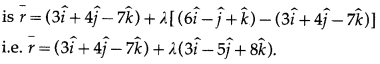Question 3.
Find the vector equation of line passing through the point having position vector $$5 \hat{i}+4 \hat{j}+3 \hat{k}$$ and having direction ratios -3, 4, 2.
Solution:
Let A be the point whose position vector is $$\bar{a}=5 \hat{i}+4 \hat{j}+3 \hat{k}$$.
Let $$\bar{b}$$ be the vector parallel to the line having direction ratios -3, 4, 2
Then, $$\bar{b}$$ = $$-3 \hat{i}+4 \hat{j}+2 \hat{k}$$
The vector equation of the line passing through A ($$\bar{a}$$) and parallel to $$\bar{b}$$ is $$\bar{r}=\bar{a}+\lambda \bar{b}$$, where λ is a scalar.
∴ the required vector equation of the line is
$$\bar{r}=5 \hat{i}+4 \hat{j}+3 \hat{k}+\lambda(-3 \hat{i}+4 \hat{j}+2 \hat{k})$$.Question 4.
Find the vector equation of the line passing through the point having position vector $$\hat{i}+2 \hat{j}+3 \hat{k}$$ and perpendicular to vectors $$\hat{i}+\hat{j}+\hat{k}$$ and $$2 \hat{i}-\hat{j}+\hat{k}$$.
Solution: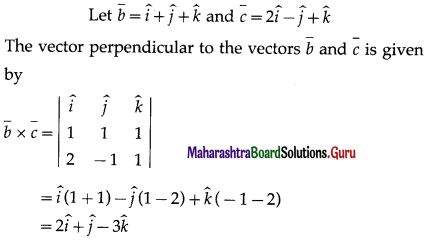Since the line is perpendicular to the vector $$\bar{b}$$ and $$\bar{c}$$, it is parallel to $$\bar{b} \times \bar{c}$$. The vector equation of the line passing through A ($$\bar{a}$$) and parallel to $$\bar{b} \times \bar{c}$$ is
$$\bar{r}=\bar{a}+\lambda(\bar{b} \times \bar{c})$$, where λ is a scalar.
Here, $$\bar{a}$$ = $$\hat{i}+2 \hat{j}+3 \hat{k}$$
Hence, the vector equation of the required line is
$$\bar{r}=(\hat{i}+2 \hat{j}+3 \hat{k})+\lambda(2 \hat{i}+\hat{j}-3 \hat{k})$$

Question 5.
Find the vector equation of the line passing through the point having position vector $$-\hat{i}-\hat{j}+2 \hat{k}$$ and parallel to the line $$\bar{r}=(\hat{i}+2 \hat{j}+3 \hat{k})+\lambda(3 \hat{i}+2 \hat{j}+\hat{k})$$.
Solution:
Let A be point having position vector $$\bar{a}$$ = $$-\hat{i}-\hat{j}+2 \hat{k}$$
The required line is parallel to the line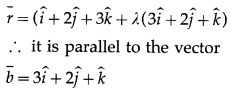The vector equation of the line passing through A($$\bar{a}$$) and parallel to $$\bar{b}$$ is $$\bar{r}$$ = $$\bar{a}$$ + λ$$\bar{b}$$ where λ is a scalar.
∴ the required vector equation of the line is
$$\overline{\mathrm{r}}=(-\hat{i}-\hat{j}+2 \hat{k})+\lambda(3 \hat{i}+2 \hat{j}+\hat{k})$$.Question 6.
Find the Cartesian equations of the line passing through A(-1, 2, 1) and having direction ratios 2, 3, 1.
Solution:
The cartesian equations of the line passing through (x1, y1, z1) and having direction ratios a, b, c are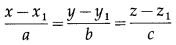∴ the cartesian equations of the line passing through the point (-1, 2, 1) and having direction ratios 2, 3, 1 are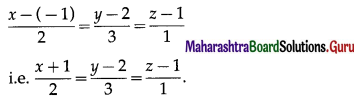Question 7.
Find the Cartesian equations of the line passing through A(2, 2, 1) and B(1, 3, 0).
Solution:
The cartesian equations of the line passing through the points (x1, y1, z1) and (x2, y2, z2) are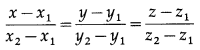Here, (x1, y1, z1) = (2, 2, 1) and (x2, y2, z2) = (1, 3, 0)
∴ the required cartesian equations are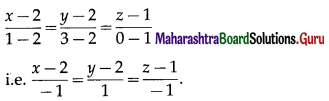Question 8.
A(-2, 3, 4), B(1, 1, 2) and C(4, -1, 0) are three points. Find the Cartesian equations of the line AB and show that points A, B, C are collinear.
Solution:
We find the cartesian equations of the line AB. The cartesian equations of the line passing through the points (x1, y1, z1) and (x2, y2, z2) are
$$\frac{x-x_{1}}{x_{2}-x_{1}}$$ = $$\frac{y-y_{1}}{y_{2}-y_{1}}$$ = $$\frac{z-z_{1}}{z_{2}-z_{1}}$$
Here, (x1, y1, z1) = (-2, 3, 4) and (x2, y2, z2) = (4, -1, 0)
∴ the required cartesian equations of the line AB are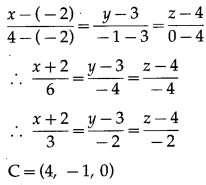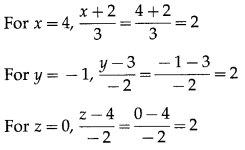∴ coordinates of C satisfy the equations of the line AB.
∴ C lies on the line passing through A and B.
Hence, A, B, C are collinear.

Question 9.
Show that lines $$\frac{x+1}{-10}=\frac{y+3}{-1}=\frac{z-4}{1}$$ and $$\frac{x+10}{-1}=\frac{y+1}{-3}=\frac{z-1}{4}$$ intersect each other. Find the co-ordinates of their point of intersection.
Solution:
The equations of the lines are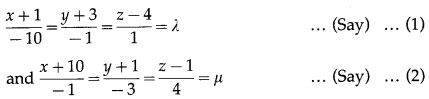From (1), x = -1 -10λ, y = -3 – 2, z = 4 + λ
∴ the coordinates of any point on the line (1) are
(-1 – 10λ, – 3 – λ, 4 + λ)
From (2), x = -10 – u, y = -1 – 3u, z = 1 + 4u
∴ the coordinates of any point on the line (2) are
(-10 – u, -1 – 3u, 1 + 4u)
Lines (1) and (2) intersect, if
(- 1 – 10λ, – 3 – λ, 4 + 2) = (- 10 – u, -1 – 3u, 1 + 4u)
∴ the equations -1 – 10λ = -10 – u, -3 – 2= – 1 – 3u
and 4 + λ = 1 + 4u are simultaneously true.
Solving the first two equations, we get, λ = 1 and u = 1. These values of λ and u satisfy the third equation also.
∴ the lines intersect.
Putting λ = 1 in (-1 – 10λ, -3 – 2, 4 + 2) or u = 1 in (-10 – u, -1 – 3u, 1 + 4u), we get
the point of intersection (-11, -4, 5).Question 10.
A line passes through (3, -1, 2) and is perpendicular to lines $$\bar{r}=(\hat{i}+\hat{j}-\hat{k})+\lambda(2 \hat{i}-2 \hat{j}+\hat{k})$$ and $$\bar{r}=(2 \hat{i}+\hat{j}-3 \hat{k})+\mu(\hat{i}-2 \hat{j}+2 \hat{k})$$. Find its equation.
Solution: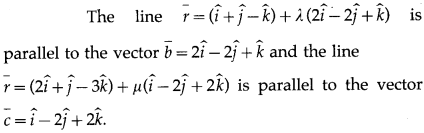The vector perpendicular to the vectors $$\bar{b}$$ and $$\bar{c}$$ is given by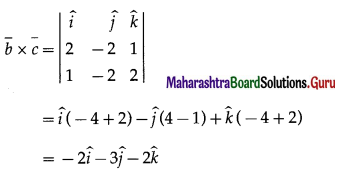Since the required line is perpendicular to the given lines, it is perpendicular to both $$\bar{b}$$ and $$\bar{c}$$.
∴ it is parallel to $$\bar{b} \times \bar{c}$$
The equation of the line passing through A($$\bar{a}$$) and parallel to $$\bar{b} \times \bar{c}$$ is
$$\bar{r}=\bar{a}+\lambda(\bar{b} \times \bar{c})$$, where λ is a scalar.
Here, $$\bar{a}$$ = $$3 \hat{i}-\hat{j}+2 \hat{k}$$
∴ the equation of the required line is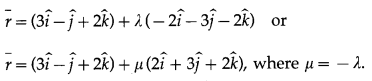Question 11.
Show that the line $$\frac{x-2}{1}=\frac{y-4}{2}=\frac{z+4}{-2}$$ passes through the origin.
Solution:
The equation of the line is
$$\frac{x-2}{1}=\frac{y-4}{2}=\frac{z+4}{-2}$$
The coordinates of the origin O are (0, 0, 0)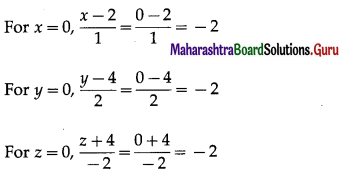∴ coordinates of the origin O satisfy the equation of the line.
Hence, the line passes through the origin.